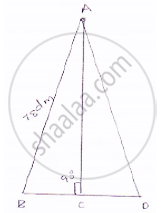Share
Notifications

View all notifications
Books Shortlist
Your shortlist is empty

# The Perimeter of a Triangular Field is 240 Dm. If Two of Its Sides Are 78 Dm and 50 Dm, Find the Length of the Perpendicular on the Side of Length 50 Dm from the Opposite Vertex. - CBSE Class 9 - Mathematics

Login
Create free account

Forgot password?
ConceptArea of a Triangle by Heron’S Formula

#### Question

The perimeter of a triangular field is 240 dm. If two of its sides are 78 dm and 50 dm, find the length of the perpendicular on the side of length 50 dm from the opposite vertex.

#### Solution

ABC be the triangle, Here a = 78 dm = AB,
BC = b = 50 dmNow, perimeter = 240 dm
⇒ AB + BC + CA = 240 dm
⇒ AC = 240 – BC – AB
⇒ AC = 112 dm
Now, 2s = AB + BC + CA
⇒ 2s = 240
⇒ s = 120 dm

∴ Area of ΔABC =sqrt(s(s-a)(s-b)(s-b)) by heron's formula

=sqrt(120(120-78)(120-50)(120-112))

=sqrt(120xx42xx70xx8)

1680 dm^2

𝐿𝑒𝑡 𝐴𝐷 𝑏𝑒 𝑝𝑒𝑟𝑝𝑒𝑛𝑑𝑖𝑐𝑢𝑎𝑙𝑎𝑟 𝑜𝑛 𝐵𝐶

Area of ΔABC = 1/2xx ABxxBC (area of triangle=(1/2xxbxxh)

=1/2xx ADxxBC=1680

⇒AD=(2xx1680)/50=67.2dm

Is there an error in this question or solution?

#### APPEARS IN

Solution The Perimeter of a Triangular Field is 240 Dm. If Two of Its Sides Are 78 Dm and 50 Dm, Find the Length of the Perpendicular on the Side of Length 50 Dm from the Opposite Vertex. Concept: Area of a Triangle by Heron’S Formula.
S